#### Dispersion definition

The dispersion5.6of a finite setof samples in a metric space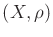is5.7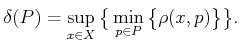(5.19)

Figure 5.4 gives an interpretation of the definition for two different metrics. An alternative way to consider dispersion is as the radius of the largest empty ball (for the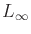metric, the balls are actually cubes). Note that at the boundary of(if it exists), the empty ball becomes truncated because it cannot exceed the boundary. There is also a nice interpretation in terms of Voronoi diagrams. Figure 5.3 can be used to help explain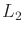dispersion in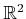. The Voronoi vertices are the points at which three or more Voronoi regions meet. These are points infor which the nearest sample is far. An open, empty disc can be placed at any Voronoi vertex, with a radius equal to the distance to the three (or more) closest samples. The radius of the largest disc among those placed at all Voronoi vertices is the dispersion. This interpretation also extends nicely to higher dimensions.

Steven M LaValle 2020-08-14